Technical Information Site of Power Supply Design

2018.05.10 DC/DC

# Resistance and Inductance of Copper Foil

DC/DC Converter PCB Layout

PCB layout is important, but in addition to layout, it is also essential to understand the PC board and the copper foil layers themselves. This time, we will explain characteristics related to the board construction and materials, as well as the resistance and inductance of copper foil.

The graphic on the right is a schematic diagram of the cross-section of a PCB. These are the basic construction and characteristics of a board, and so they should be remembered. The main points are listed below.

• Often the copper foil layers on the top and bottom surfaces are different in thickness from those on the inner layers.
• Often the copper foil layers on the core material are thicker, to increase heat dissipation.
• Core materials have general-use thicknesses, with the thickness adjusted using pre-preg.
• There are materials in which migration may occur, depending on the core material and the pre-preg type, and in some cases such materials cannot withstand high-humidity testing.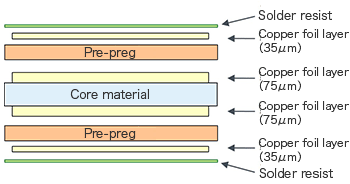Resistance of Copper Foil

Of course, copper foil (wiring) has electrical resistance. Under conditions in which a large current flows, conduction losses, that is, a voltage drop and heat generation, occur. Hence where large-current lines are concerned, it is important to review the resistance values of copper foil layers.

The resistance of copper foil is described as a value per unit area. Figure 10 indicates the resistance values per unit area of copper foil. The resistance values are for the generally application conditions of a copper foil thickness of 35 μm, width 1 mm, and length 1 mm.

The following equation is used for general calculations of resistance.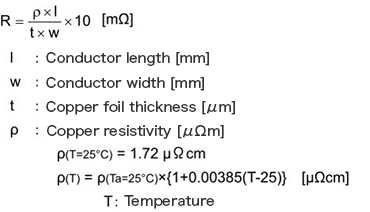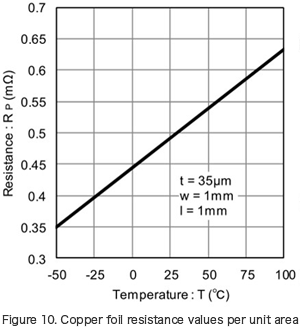When calculating from the resistance value RP per unit area read off from Figure 10, we obtain the following.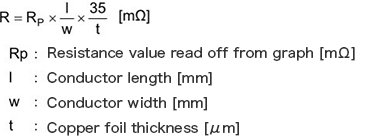For example, at 25°C, the resistance value of copper foil of width 3 mm and length 50 mm, calculated as follows, is 8.17 mΩ.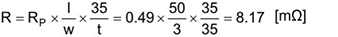From this resistance value, the voltage drop when a 3 A current flows is 24.5 mV. If the temperature rises to 100°C, from the graph we see that the resistance value increases 29%. Hence the voltage drop also increases, to 31.6 mV.

This voltage drop due to the copper foil can be a major problem, depending on circumstances. In essence, the wiring width should be studied in view of the current and temperature conditions.

Inductance of Copper Foil

As is also natural, copper foil has an inductance as well. It should be born in mind that parasitic components for resistance, capacitance, and inductance are always present.

The inductance of copper foil is expressed by the following equation.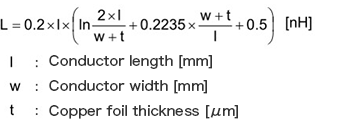From this equation, we see that the inductance of copper foil depends hardly at all on the foil thickness.

Figure 11 shows calculated values for copper foil inductance. As is clear from the graph, there is surprisingly little decrease in the inductance even when the line width is doubled.

In order to suppress the effects of parasitic inductance, the best approach is to shorten wiring lengths.

If the current propagating in printed wiring with inductance L (H) changes by i (A) in time t (s), then the following voltage appears across the ends of the printed wiring.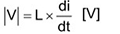For example, if a 2 A current flows for 10 ns in printed wiring with a parasitic inductance of 6 nH, then the following voltage occurs.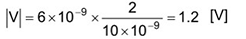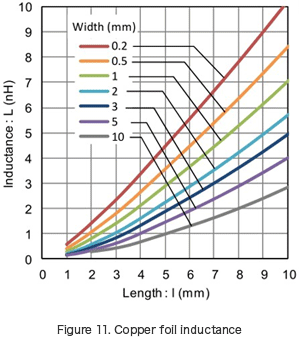Parasitic inductance can also cause large voltages, depending on the circumstances, and not only circuit operation can be affected, but components may be damaged or destroyed, so proper precautions must be taken.

#### Key Points:

・Understand the basic construction of a printed circuit board.

・The resistance of copper foil layers appears as a voltage drop, and is dependent on temperature.

・The inductance of copper foil layers can in some cases generate high voltages, so care should be exercised.

・Shortening wiring lengths is effective for reducing inductance.

This website uses cookies.

By continuing to browse this website without changing your web-browser cookie settings, you are agreeing to our use of cookies.